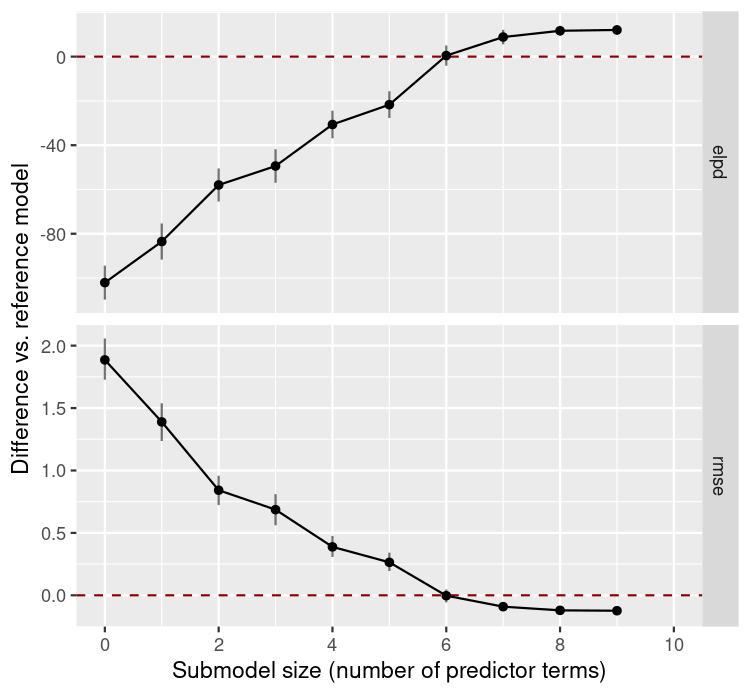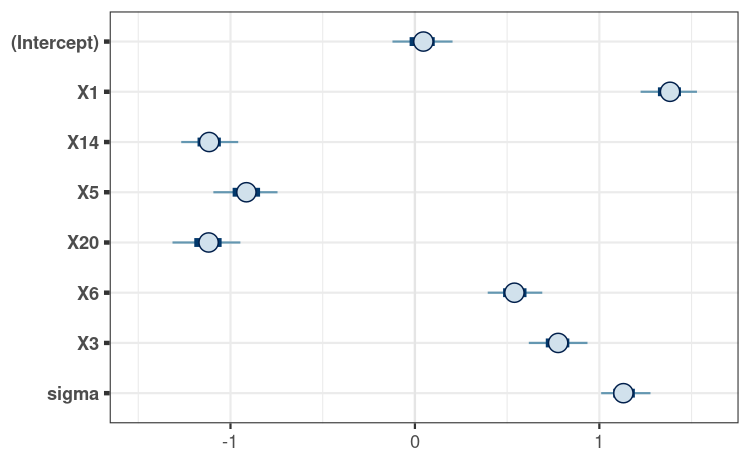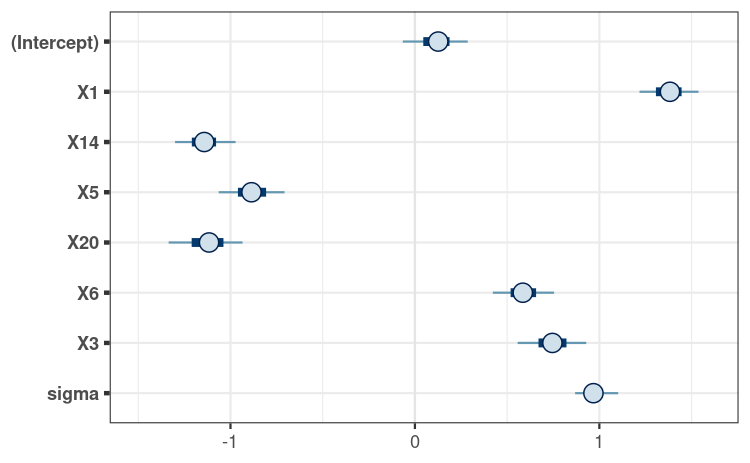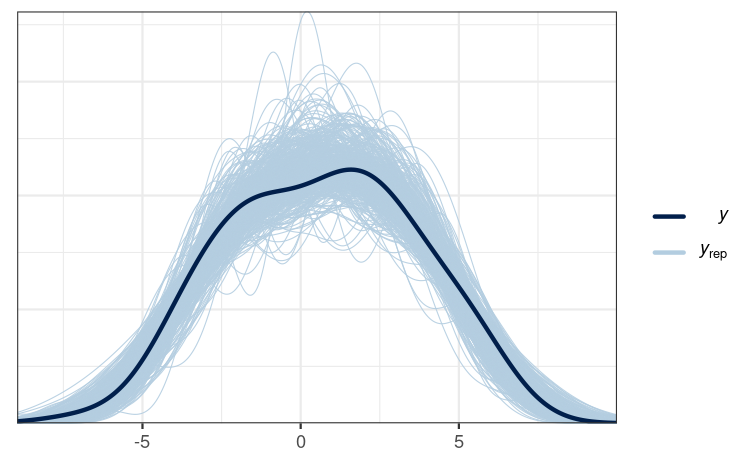# projpred: Projection predictive feature selection

## Introduction

This vignette shows the main functionalities of the projpred package, which implements the projection predictive variable selection for various regression models (see section “Supported types of models” below for more details on supported model types). What is special about the projection predictive variable selection is that it not only performs a variable selection, but also allows for valid post-selection inference.

The projection predictive variable selection is based on the ideas of Goutis and Robert (1998) and Dupuis and Robert (2003). The methods implemented in projpred are described in detail in Piironen, Paasiniemi, and Vehtari (2020) and Catalina, Bürkner, and Vehtari (2022). They are evaluated in comparison to many other methods in Piironen and Vehtari (2017a). For details on how to cite projpred, see the projpred citation info on CRAN.1

## Data

For this vignette, we use projpred’s df_gaussian data. It contains 100 observations of 20 continuous predictor variables X1, …, X20 (originally stored in a sub-matrix; we turn them into separate columns below) and one continuous response variable y.

data("df_gaussian", package = "projpred")
dat_gauss <- data.frame(y = df_gaussian$y, df_gaussian$x)

## Reference model

First, we have to construct a reference model for the projection predictive variable selection. This model is considered as the best (“reference”) solution to the prediction task. The aim of the projection predictive variable selection is to find a subset of a set of candidate predictors which is as small as possible but achieves a predictive performance as close as possible to that of the reference model.

Usually (and this is also the case in this vignette), the reference model will be an rstanarm or brms fit. To our knowledge, rstanarm and brms are currently the only packages for which a get_refmodel() method (which establishes the compatibility with projpred) exists. Creating a reference model object via one of these get_refmodel.stanreg() or brms::get_refmodel.brmsfit() methods (either implicitly by a call to a top-level function such as project(), varsel(), and cv_varsel(), as done below, or explicitly by a call to get_refmodel()) leads to a “typical” reference model object. In that case, all candidate models are actual submodels of the reference model. In general, however, this assumption is not necessary for a projection predictive variable selection (see, e.g., Piironen, Paasiniemi, and Vehtari 2020). This is why “custom” (i.e., non-“typical”) reference model objects allow to avoid this assumption (although the candidate models of a “custom” reference model object will still be actual submodels of the full formula used by the search procedure—which does not have to be the same as the reference model’s formula, if the reference model possesses a formula at all). Such “custom” reference model objects can be constructed via init_refmodel() (or get_refmodel.default()), as shown in section “Examples” of the ?init_refmodel help.2

Here, we use the rstanarm package to fit the reference model. If you want to use the brms package, simply replace the rstanarm fit (of class stanreg) in all the code below by your brms fit (of class brmsfit). Only note that in case of a brms fit, we recommend to specify argument brms_seed of brms::get_refmodel.brmsfit().

library(rstanarm)

For our rstanarm reference model, we use the Gaussian distribution as the family for our response. With respect to the predictors, we only include the linear main effects of all 20 predictor variables. Compared to the more complex types of reference models supported by projpred (see section “Supported types of models” below), this is a quite simple reference model which is sufficient, however, to demonstrate the interplay of projpred’s functions.

We use rstanarm’s default priors in our reference model, except for the regression coefficients for which we use a regularized horseshoe prior with the hyperprior for its global shrinkage parameter following Piironen and Vehtari (2017b) and Piironen and Vehtari (2017c). In R code, these are the preparation steps for the regularized horseshoe prior:

# Number of regression coefficients:
( D <- sum(grepl("^X", names(dat_gauss))) )
 20
# Prior guess for the number of relevant (i.e., non-zero) regression
# coefficients:
p0 <- 5
# Number of observations:
N <- nrow(dat_gauss)
# Hyperprior scale for tau, the global shrinkage parameter (note that for the
# Gaussian family, 'rstanarm' will automatically scale this by the residual
# standard deviation):
tau0 <- p0 / (D - p0) * 1 / sqrt(N)

We now fit the reference model to the data. To make this vignette build faster, we use only 2 MCMC chains and 500 iterations per chain (with half of them being discarded as warmup draws). In practice, 4 chains and 2000 iterations per chain are reasonable defaults. Furthermore, we make use of rstan’s parallelization, which means to run each chain on a separate CPU core.3 If you run the following code yourself, you can either rely on an automatic mechanism to detect the number of CPU cores (like the parallel::detectCores() function shown below) or adapt ncores manually to your system.

# Set this manually if desired:
ncores <- parallel::detectCores(logical = FALSE)
### Only for technical reasons in this vignette (you can omit this when running
### the code yourself):
ncores <- min(ncores, 2L)
###
options(mc.cores = ncores)
refm_fit <- stan_glm(
y ~ X1 + X2 + X3 + X4 + X5 + X6 + X7 + X8 + X9 + X10 + X11 + X12 + X13 + X14 +
X15 + X16 + X17 + X18 + X19 + X20,
family = gaussian(),
data = dat_gauss,
prior = hs(global_scale = tau0),
### Only for the sake of speed (not recommended in general):
chains = 2, iter = 500,
###
seed = 2052109, QR = TRUE, refresh = 0
)

Usually, we would now have to check the convergence diagnostics (see, e.g., ?posterior::diagnostics and ?posterior::default_convergence_measures). However, due to the technical reasons for which we reduced chains and iter, we skip this step here.

## Variable selection

Now, projpred comes into play.

library(projpred)

In projpred, the projection predictive variable selection consists of a search part and an evaluation part. The search part determines the solution path, i.e., the best submodel for each submodel size (number of predictor terms). The evaluation part determines the predictive performance of the submodels along the solution path.

There are two functions for performing the variable selection: varsel() and cv_varsel(). In contrast to varsel(), cv_varsel() performs a cross-validation (CV) by running the search part with the training data of each CV fold separately (an exception is validate_search = FALSE, see ?cv_varsel and below) and running the evaluation part on the corresponding test set of each CV fold. Because of this CV, cv_varsel() is recommended over varsel(). Thus, we use cv_varsel() here. Nonetheless, running varsel() first can offer a rough idea of the performance of the submodels (after projecting the reference model onto them). A more principled projpred workflow is work under progress.

Here, we use only some of the available arguments; see the documentation of cv_varsel() for the full list of arguments. By default, cv_varsel() runs a leave-one-out (LOO) CV (see argument cv_method) which also cross-validates the search (see argument validate_search). Here, we set argument validate_search to FALSE to obtain rough preliminary results and make this vignette build faster. If possible (in terms of computation time), we recommend using the default of validate_search = TRUE to avoid overfitting in the selection of the submodel size. Here, we also set nclusters_pred to a low value of 20 only to speed up the building of the vignette. By modifying argument nterms_max, we impose a limit on the submodel size until which the search is continued. Typically, one has to run the variable selection with a large nterms_max first (the default value may not even be large enough) and only after inspecting the results from this first run, one is able to set a reasonable nterms_max in subsequent runs. The value we are using here (9) is based on such a first run (which is not shown here, though).

cvvs <- cv_varsel(
refm_fit,
### Only for the sake of speed (not recommended in general):
validate_search = FALSE,
nclusters_pred = 20,
###
nterms_max = 9,
seed = 411183
)

The first step after running the variable selection should be the decision for a final submodel size. This should be the first step (in particular, before inspecting the solution path) in order to avoid a user-induced selection bias (which could occur if the user made the submodel size decision dependent on the solution path). To decide for a submodel size, there are several performance statistics we can plot as a function of the submodel size. Here, we use the expected log (pointwise) predictive density (for a new dataset) (ELPD; empirically, this is the sum of the log predictive densities of the observations in the evaluation—or “test”—set) and the root mean squared error (RMSE). By default, the performance statistics are plotted on their original scale, but with deltas = TRUE, they are calculated as differences from a baseline model (which is the reference model by default, at least in the most common cases). Since the differences are usually of more interest (with regard to the submodel size decision), we directly plot with deltas = TRUE here (note that as validate_search = FALSE, this result is slightly optimistic, and the plot looks different when validate_search = TRUE is used):

plot(cvvs, stats = c("elpd", "rmse"), deltas = TRUE, seed = 54548)Based on that plot (see ?plot.vsel for a description), we would decide for a submodel size of 6 because that’s the point where the performance measures level off and are close enough to the reference model’s performance (note that since the plot is affected by validate_search = FALSE, this manual decision based on the plot is affected, too):

modsize_decided <- 6

Note that projpred offers the suggest_size() function which may help in the decision for a submodel size, but this is a rather heuristic method and needs to be interpreted with caution (see ?suggest_size):

suggest_size(cvvs)
 6

Here, we would get the same final submodel size (6) as by our manual decision (suggest_size() is also affected by validate_search = FALSE). Note that by default, suggest_size() uses the ELPD as performance statistic.

Only now, after we have made a decision for the submodel size, we inspect further results from the variable selection and, in particular, the solution path. For example, we can simply print() the resulting object:

cvvs

Family: gaussian

Formula: y ~ X1 + X2 + X3 + X4 + X5 + X6 + X7 + X8 + X9 + X10 + X11 +
X12 + X13 + X14 + X15 + X16 + X17 + X18 + X19 + X20
Observations: 100
CV method: LOO search not included
Search method: l1, maximum number of terms 9
Number of clusters used for selection: 1
Number of clusters used for prediction: 20
Suggested Projection Size: 6

Selection Summary:
size solution_terms elpd.loo  se   diff diff.se
0           <NA>   -249.2 5.6 -102.1     7.7
1             X1   -230.6 6.1  -83.5     8.2
2            X14   -205.0 6.2  -58.0     7.5
3             X5   -196.5 7.2  -49.4     7.6
4            X20   -177.7 5.9  -30.6     6.3
5             X6   -168.8 5.0  -21.7     6.0
6             X3   -146.6 5.1    0.5     4.6
7             X8   -138.2 4.5    8.9     3.3
8            X11   -135.4 4.5   11.7     2.3
9            X10   -135.0 4.4   12.1     2.2
### Alternative modifying the number of printed decimal places:
# print(cvvs, digits = 2)
### 

The solution path can be seen in the print() output (column solution_terms), but it is also accessible through the solution_terms() function:

( soltrms <- solution_terms(cvvs) )
 "X1"  "X14" "X5"  "X20" "X6"  "X3"  "X8"  "X11" "X10"

Combining the decided submodel size of 6 with the solution path leads to the following terms (as well as the intercept) as the predictor terms of the final submodel:

( soltrms_final <- head(soltrms, modsize_decided) )
 "X1"  "X14" "X5"  "X20" "X6"  "X3" 

## Post-selection inference

The project() function returns an object of class projection which forms the basis for convenient post-selection inference. By the following code, project() will project the reference model onto the final submodel once again4:

prj <- project(refm_fit, solution_terms = soltrms_final)

For more accurate results, we could have increased argument ndraws of project() (up to the number of posterior draws in the reference model). This increases the runtime, which we don’t want in this vignette.

Next, we create a matrix containing the projected posterior draws stored in the depths of project()’s output:

prj_mat <- as.matrix(prj)

This matrix is all we need for post-selection inference. It can be used like any matrix of draws from MCMC procedures, except that it doesn’t reflect a typical posterior distribution, but rather a projected posterior distribution, i.e., the distribution arising from the deterministic projection of the reference model’s posterior distribution onto the parameter space of the final submodel.

### Marginals of the projected posterior

The posterior package provides a general way to deal with posterior distributions, so it can also be applied to our projected posterior. For example, to calculate summary statistics for the marginals of the projected posterior:

library(posterior)
prj_drws <- as_draws_matrix(prj_mat)
# In the following call, as.data.frame() is used only because pkgdown
# versions > 1.6.1 don't print the tibble correctly.
as.data.frame(summarize_draws(
prj_drws,
"median", "mad", function(x) quantile(x, probs = c(0.025, 0.975))
))
     variable     median        mad       2.5%      97.5%
1 (Intercept)  0.0458985 0.10183583 -0.1505310  0.2205816
2          X1  1.3834312 0.09130185  1.1956560  1.5532896
3         X14 -1.1157218 0.09405313 -1.2834051 -0.9318850
4          X5 -0.9136915 0.11058452 -1.1096153 -0.7263205
5         X20 -1.1185014 0.10942521 -1.3460276 -0.9092710
6          X6  0.5395952 0.09545216  0.3489350  0.7149177
7          X3  0.7767160 0.09659702  0.5948437  0.9772419
8       sigma  1.1304147 0.08527177  0.9919125  1.3107667

A visualization of the projected posterior can be achieved with the bayesplot package, for example using its mcmc_intervals() function:

library(bayesplot)
bayesplot_theme_set(ggplot2::theme_bw())
mcmc_intervals(prj_mat) +
ggplot2::coord_cartesian(xlim = c(-1.5, 1.6))Note that we only visualize the 1-dimensional marginals of the projected posterior here. To gain a more complete picture, we would have to visualize at least some 2-dimensional marginals of the projected posterior (i.e., marginals for pairs of parameters).

For comparison, consider the marginal posteriors of the corresponding parameters in the reference model:

refm_mat <- as.matrix(refm_fit)
mcmc_intervals(refm_mat, pars = colnames(prj_mat)) +
ggplot2::coord_cartesian(xlim = c(-1.5, 1.6))Here, the reference model’s marginal posteriors differ only slightly from the marginals of the projected posterior. This does not necessarily have to be the case.

### Predictions

Predictions from the final submodel can be made by proj_linpred() and proj_predict().

We start with proj_linpred(). For example, suppose we have the following new observations:

( dat_gauss_new <- setNames(
as.data.frame(replicate(length(soltrms_final), c(-1, 0, 1))),
soltrms_final
) )
  X1 X14 X5 X20 X6 X3
1 -1  -1 -1  -1 -1 -1
2  0   0  0   0  0  0
3  1   1  1   1  1  1

Then proj_linpred() can calculate the linear predictors5 for all new observations from dat_gauss_new. Depending on argument integrated, these linear predictors can be averaged across the projected draws (within each new observation). For instance, the following computes the expected values of the new observations’ predictive distributions:6

prj_linpred <- proj_linpred(prj, newdata = dat_gauss_new, integrated = TRUE)
cbind(dat_gauss_new, linpred = as.vector(prj_linpred$pred))  X1 X14 X5 X20 X6 X3 linpred 1 -1 -1 -1 -1 -1 -1 0.50053930 2 0 0 0 0 0 0 0.04160798 3 1 1 1 1 1 1 -0.41732334 If dat_gauss_new also contained response values (i.e., y values in this example), then proj_linpred() would also evaluate the log predictive density at these. With proj_predict(), we can obtain draws from predictive distributions based on the final submodel. In contrast to proj_linpred(<...>, integrated = FALSE), this encompasses not only the uncertainty arising from parameter estimation, but also the uncertainty arising from the observational (or “sampling”) model for the response.7 This is useful for what is usually termed a posterior predictive check (PPC), but would have to be termed something like a posterior-projection predictive check (PPPC) here: prj_predict <- proj_predict(prj, .seed = 762805) # Using the 'bayesplot' package: ppc_dens_overlay(y = dat_gauss$y, yrep = prj_predict, alpha = 0.9, bw = "SJ")This PPPC shows that our final projection is able to generate predictions similar to the observed response values, which indicates that this model is reasonable, at least in this regard.

## Supported types of models

In principle, the projection predictive variable selection requires only little information about the form of the reference model. Although many aspects of the reference model coincide with those from the submodels if a “typical” reference model object is used, this does not need to be the case if a “custom” reference model object is used (see section “Reference model” above for the definition of “typical” and “custom” reference model objects). This explains why in general, the following remarks refer to the submodels and not to the reference model.

Apart from the gaussian() response family used in this vignette, projpred also supports the binomial()8 and the poisson() family. On the side of the predictors, projpred not only supports linear main effects as shown in this vignette, but also interactions, multilevel9, and—as an experimental feature—additive10 terms.

Transferring this vignette (which employs a “typical” reference model) to such more complex problems is straightforward: Basically, only the code for fitting the reference model via rstanarm or brms needs to be adapted. The projpred code stays almost the same. Only note that in case of multilevel or additive reference models, some projpred functions then have slightly different options for a few arguments. See the documentation for details.

For example, to apply projpred to the VerbAgg dataset from the lme4 package, a corresponding multilevel reference model for the binary response r2 could be created by the following code:

data("VerbAgg", package = "lme4")
refm_fit <- stan_glmer(
r2 ~ btype + situ + mode + (btype + situ + mode | id),
family = binomial(),
data = VerbAgg,
seed = 82616169, QR = TRUE, refresh = 0
)

As an example for an additive (non-multilevel) reference model, consider the lasrosas.corn dataset from the agridat package. A corresponding reference model for the continuous response yield could be created by the following code (note that pp_check(refm_fit) gives a bad PPC in this case, so there’s still room for improvement):

data("lasrosas.corn", package = "agridat")
# Convert year to a factor (this could also be solved by using
# factor(year) in the formula, but we avoid that here to put more emphasis on
# the demonstration of the smooth term):
lasrosas.corn$year <- as.factor(lasrosas.corn$year)
refm_fit <- stan_gamm4(
yield ~ year + topo + t2(nitro, bv),
family = gaussian(),
data = lasrosas.corn,
seed = 4919670, QR = TRUE, refresh = 0
)

As an example for an additive multilevel reference model, consider the gumpertz.pepper dataset from the agridat package. A corresponding reference model for the binary response disease could be created by the following code:

data("gumpertz.pepper", package = "agridat")
refm_fit <- stan_gamm4(
disease ~ field + leaf + s(water),
random = ~ (1 | row) + (1 | quadrat),
family = binomial(),
data = gumpertz.pepper,
seed = 14209013, QR = TRUE, refresh = 0
)

## Troubleshooting

Sometimes, the ordering of the predictor terms in the solution path makes sense, but for increasing submodel size, the performance measures of the submodels do not approach that of the reference model. There are different reasons that can explain this behavior (the following list might not be exhaustive, though):

1. The reference model’s posterior may be so wide that the default ndraws_pred could be too small. Usually, this comes in combination with a difference in predictive performance which is comparatively small. Increasing ndraws_pred should help, but it also increases the computational cost. Re-fitting the reference model and thereby ensuring a narrower posterior (usually by employing a stronger sparsifying prior) should have a similar effect.
2. For non-Gaussian models, the discrepancy may be due to the fact that the penalized iteratively reweighted least squares (PIRLS) algorithm might have convergence issues . In this case, the latent-space approach by Catalina, Bürkner, and Vehtari (2021) might help.
3. If you are using varsel(), then the lack of the CV in varsel() may lead to overconfident and overfitted results. In this case, try running cv_varsel() instead of varsel() (which you should in any case for your final results).

1. The citation information can be accessed offline by typing print(citation("projpred"), bibtex = TRUE) within R.↩︎

2. We will cover custom reference models more deeply in a future vignette.↩︎

3. More generally, the number of chains is split up as evenly as possible among the number of CPU cores.↩︎

4. During the forward search, the reference model has already been projected onto all candidate models (this was where arguments ndraws and nclusters of cv_varsel() came into play). During the evaluation of the submodels along the solution path, the reference model has already been projected onto those submodels (this was where arguments ndraws_pred and nclusters_pred of cv_varsel() came into play). In principle, one could use the results from the evaluation part for post-selection inference, but due to a bug in the current implementation (see GitHub issue #168), we currently have to project once again.↩︎

5. proj_linpred() can also transform the linear predictor to response scale, but here, this is the same as the linear predictor scale (because of the identity link function).↩︎

6. Beware that this statement is correct here because of the Gaussian family with the identity link function. For other families (which usually come in combination with a different link function), one would typically have to use transform = TRUE in order to make this statement correct.↩︎

7. In case of the Gaussian family we are using here, the uncertainty arising from the observational model is the uncertainty due to the residual standard deviation.↩︎

8. Via brms::get_refmodel.brmsfit(), the brms::bernoulli() family is supported as well.↩︎

9. Multilevel models are also known as hierarchical models or models with partially pooled, group-level, or—in frequentist terms—random effects.↩︎

10. Additive terms are also known as smooth terms.↩︎